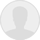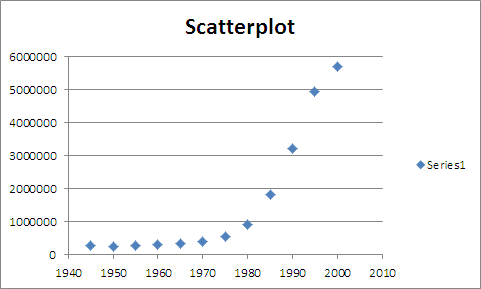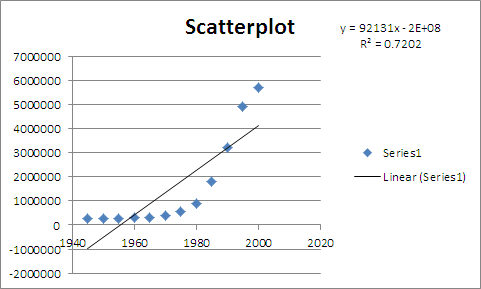# 2023 Time series are particularly useful to track variables such as revenues costs and profits over time Time series models help

Time Series Modeling 2023 Business Finance

2023 Time series are particularly useful to track variables such as revenues costs and profits over time Time series models help

Time series are particularly useful to track variables such as revenues, costs, and profits over time. Time series models help evaluate performance and make predictions. Consider the following and respond in a minimum of 175 words:

• Time series decomposition seeks to separate the time series (Y) into 4 components: trend (T), cycle (C), seasonal (S), and irregular (I). What is the difference between these components?
• The model can be additive or multiplicative.When we do use an additive model? When do we use a multiplicative model?
• The following list gives the gross federal debt(in millions of dollars) for the U.S. every 5 years from 1945 to 2000:

Year    Gross Federal Debt (\$millions)

1945    260,123

1950    256,853

1955    274,366

1960    290,525

1965    322,318

1970    380,921

1975    541,925

1980    909,050

1985    1,817,521

1990    3,206,564

1995    4,921,005

2000    5,686,338

• Construct a scatter plot with this data. Do you observe a trend? If so, what type of trend do you observe?
• Use Excel to fit a linear trend and an exponential trend to the data. Display the models and their respective r^2.
• Interpret both models. Which model seems to be more appropriate? Why?

•Mayuriyedave answered thisWas this answer helpful?001,168 answersscatteplot of data is,